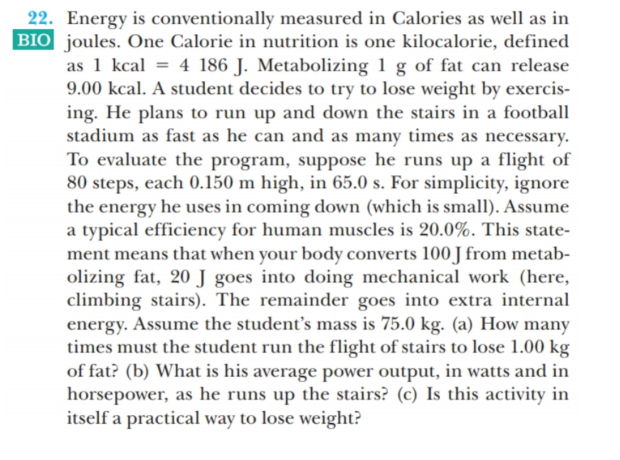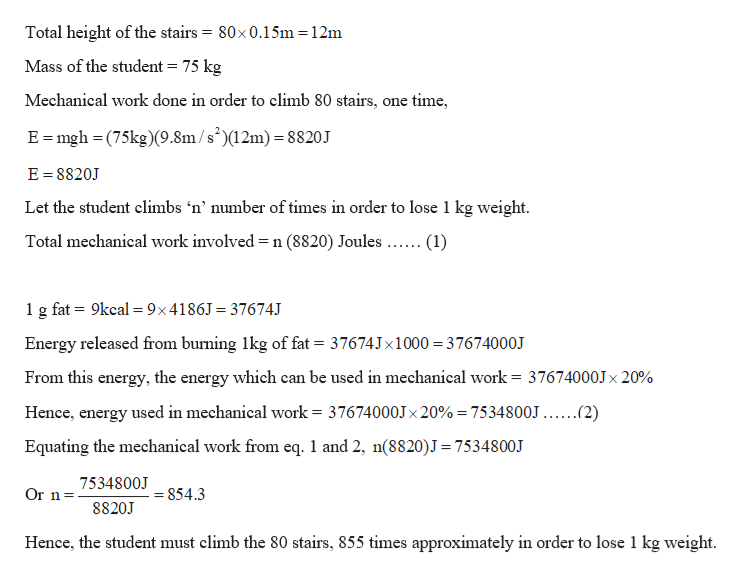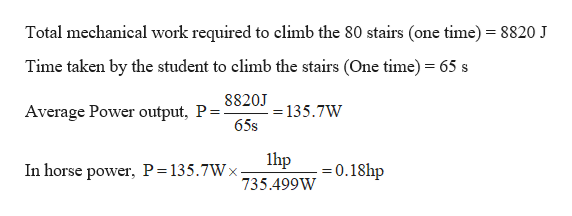# 22. Energy is conventionally measured in Calories as well as inBIO joules. One Calorie in nutrition is one kilocalorie, definedas 1 kcal = 4 186 J. Metabolizing 1 g of fat can release9.00 kcal. A student decides to try to lose weight by exercis-ing. He plans to run up and down the stairs in a footballstadium as fast as he can and as many times as necessary.To evaluate the program, suppose he runs up a flight of80 steps, each 0.150 m high, in 65.0 s. For simplicity, ignorethe energy he uses in coming down (which is small). Assumea typical efficiency for human muscles is 20.0%. This state-ment means that when your body converts 100 J from metab-olizing fat, 20 J goes into doing mechanical work (here,climbing stairs). The remainder goes into extra internalenergy. Assume the student's mass is 75.0 kg. (a) How manytimes must the student run the flight of stairs to lose 1.00 kgof fat? (b) What is his average power output, in watts and inhorsepower, as he runs up the stairs? (c) Is this activity initself a practical way to lose weight?

Question
1 viewshelp_outlineImage Transcriptionclose22. Energy is conventionally measured in Calories as well as in BIO joules. One Calorie in nutrition is one kilocalorie, defined as 1 kcal = 4 186 J. Metabolizing 1 g of fat can release 9.00 kcal. A student decides to try to lose weight by exercis- ing. He plans to run up and down the stairs in a football stadium as fast as he can and as many times as necessary. To evaluate the program, suppose he runs up a flight of 80 steps, each 0.150 m high, in 65.0 s. For simplicity, ignore the energy he uses in coming down (which is small). Assume a typical efficiency for human muscles is 20.0%. This state- ment means that when your body converts 100 J from metab- olizing fat, 20 J goes into doing mechanical work (here, climbing stairs). The remainder goes into extra internal energy. Assume the student's mass is 75.0 kg. (a) How many times must the student run the flight of stairs to lose 1.00 kg of fat? (b) What is his average power output, in watts and in horsepower, as he runs up the stairs? (c) Is this activity in itself a practical way to lose weight? fullscreen
check_circle

Step 1

Given:

Mass of the student = 75 kg

Height of one stair = 0.15 m

Number of stairs = 80

Time taken to climb the 80 stairs = 65 s

1 g fat burns and gives energy = 9 kcal

1 kcal = 4186 J

Step 2

(A) Calculating the number of times, the student must climb the stairs in order to lose 1 kg:help_outlineImage TranscriptioncloseTotal height of the stairs = 80x0.15m = 12m Mass of the student = 75 kg Mechanical work done in order to climb 80 stairs, one time, E= mgh = (75kg)(9.8m /s)(12m) = 8820J E = 8820J Let the student climbs 'n’ number of times in order to lose 1 kg weight. Total mechanical work involved =n (8820) Joules.. (1) 1g fat = 9kcal = 9×4186J = 37674J Energy released from burning 1kg of fat = 37674J×1000 = 37674000J From this energy, the energy which can be used in mechanical work = 37674000J× 20% %3D Hence, energy used in mechanical work = 37674000J× 20% = 7534800J ...(2) %3! Equating the mechanical work from eq. 1 and 2, n(8820)J= 7534800J 7534800J Or n= = 854.3 8820J Hence, the student must climb the 80 stairs, 855 times approximately in order to lose 1 kg weight. fullscreen
Step 3

(B) Calculating the aver...help_outlineImage TranscriptioncloseTotal mechanical work required to climb the 80 stairs (one time) = 8820 J Time taken by the student to climb the stairs (One time) = 65 s 8820J Average Power output, P= 65s = 135.7W lhp =0.18hp In horse power, P=135.7W x 735.499W fullscreen

### Want to see the full answer?

See Solution

#### Want to see this answer and more?

Solutions are written by subject experts who are available 24/7. Questions are typically answered within 1 hour.*

See Solution
*Response times may vary by subject and question.
Tagged in

### Physics